# The complex ion Fe(NH3)42- is paramagnetic. The shape of this complex is a) octahedral b) tetrahedral...

The complex ion Fe(NH3)42- is paramagnetic. The shape of this complex is

a) octahedral

b) tetrahedral

c) Square planar

Ans : b) tetrahedral

There are four ammonia ligands bonded to central Fe atom. NH3 is a strong field ligand and since the ion is paramagnetic there are unpaired electrons in the orbital.

This indicates that the shape of ion is tetrahedral as the hybridization of Fe is sp3.

##### Add Answer of: The complex ion Fe(NH3)42- is paramagnetic. The shape of this complex is a) octahedral b) tetrahedral...
Similar Homework Help Questions
• ### All of the following complexes are either octahedral or tetrahedral. Determine if the complex is diamagnetic or par...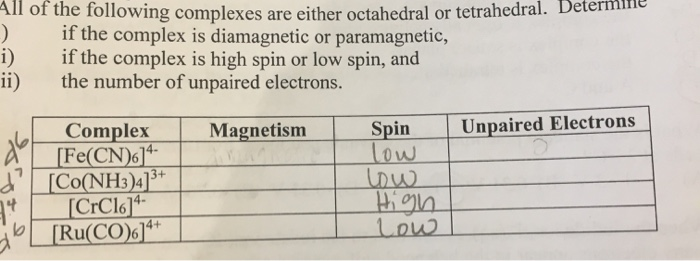All of the following complexes are either octahedral or tetrahedral. Determine if the complex is diamagnetic or paramagnetic, if the complex is high spin or low spin, and ii) the number of unpaired electrons. Unpaired Electrons Spin low Magnetism Complex [Fe(CN)6]* [Co(NH3)4]3 13+ 14- 4+ [Ru(CO)6] All of the following complexes are either octahedral or tetrahedral. Determine if the complex is diamagnetic or paramagnetic, if the complex is high spin or low spin, and ii) the number of unpaired electrons....

• ### The transition metal ion, Fe(III), can form octahedral or tetrahedral complexes depending on the ligand it...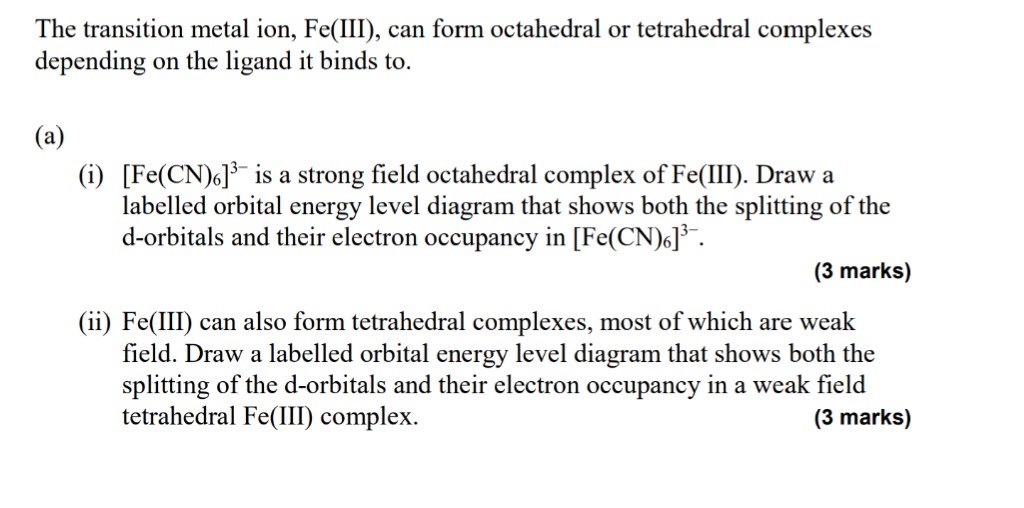The transition metal ion, Fe(III), can form octahedral or tetrahedral complexes depending on the ligand it binds to. (a) (i) [Fe(CN)6]3- is a strong field octahedral complex of Fe(III). Draw a labelled orbital energy level diagram that shows both the splitting of the d-orbitals and their electron occupancy in [Fe(CN)6]. (3 marks) (ii) Fe(III) can also form tetrahedral complexes, most of which are weak field. Draw a labelled orbital energy level diagram that shows both the splitting of the d-orbitals...

• ### due ASAp!! please help 1. 2. 3. A transition metal complex with the formula Fe(NH3)(CN)3] is...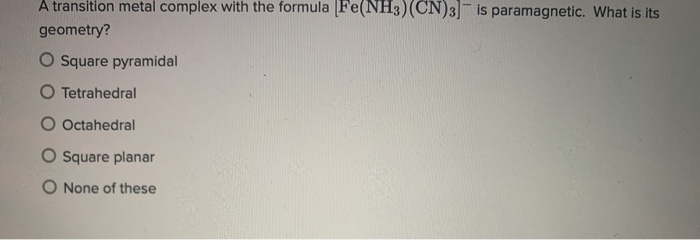due ASAp!! please help 1. 2. 3. A transition metal complex with the formula Fe(NH3)(CN)3] is paramagnetic. What is its geometry? O Square pyramidal O Tetrahedral Octahedral O Square planar O None of these Use organic nomenclature to name this molecule: Enter your answer here How many stereocenters (chiral carbon atoms) are there in this molecule? Submit your answer as a number. For example, enter 10, NOT ten. Enter your answer here Show transcribed image text

• ### tetrahedral and square planar complex

tetrahedral and square planar complex [Fe(H2O)4]2+

• ### 2.) Give the point group assignment of the following transition metal compounds: a.) trans- [PtCl2Br2]the ion...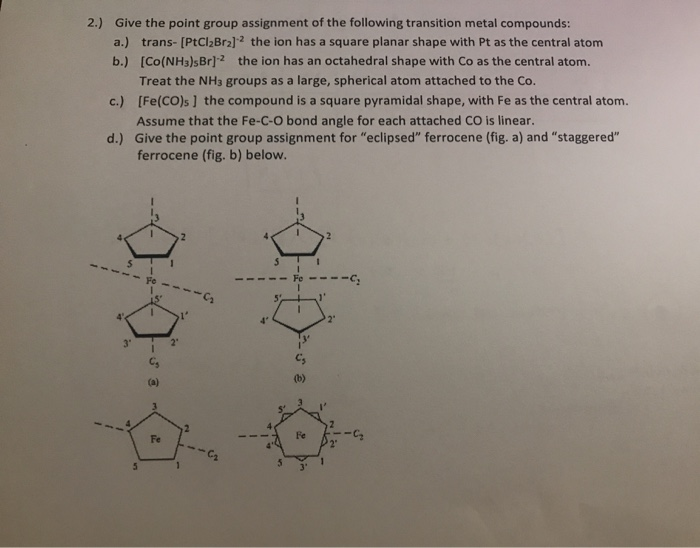2.) Give the point group assignment of the following transition metal compounds: a.) trans- [PtCl2Br2]the ion has a square planar shape with Pt as the central atom b.) [CO(NH3)sBr]? the ion has an octahedral shape with Co as the central atom. Treat the NH3 groups as a large, spherical atom attached to the Co. c.) [Fe(CO)s) the compound is a square pyramidal shape, with Fe as the central atom. Assume that the Fe-C-O bond angle for each attached CO is...

• ### Which of the following molecule has a bent molecular shape? b. BF3 e. HCN d. NH3...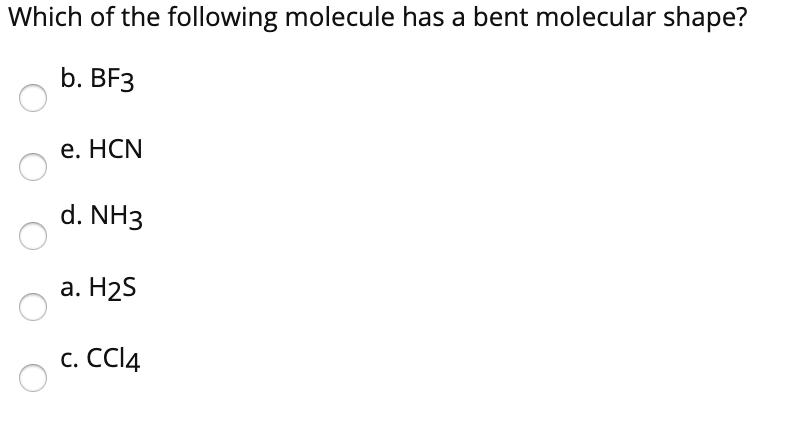Which of the following molecule has a bent molecular shape? b. BF3 e. HCN d. NH3 a. H2S C. CCl4 Identify the correct electron geometry of NH3 molecule. e. Octahedral d. Trigonal bipyramidal a. Linear b. Trigonal planar c. Tetrahedral Show transcribed image text

• ### Which one of the following octahedral complex ions is diamagnetic? Note: NH3 is a strong-field ligand....

Which one of the following octahedral complex ions is diamagnetic? Note: NH3 is a strong-field ligand. (a) [Fe(NH3)6] 3+ (b) [Fe(NH3)6] 2+ (c) [Cr(NH3)6] 3+ (d) [Cr(NH3)6] 2+ (e) [Co(NH3)6] 2+

• ### VSEPR theory predicts which shape for the molecular structure of the following molecules or molecular ions?...

VSEPR theory predicts which shape for the molecular structure of the following molecules or molecular ions? (i.e. the arrangement of only the bonded atoms about the central atom) bent (or angular) linear octahedral see-saw square planar square pyramidal tetrahedral trigonal bipyramidal trigonal planar trigonal pyramidal T-shaped  ICl3 molecule    (The central atom is I.) bent (or angular) linear octahedral see-saw square planar square pyramidal tetrahedral trigonal bipyramidal trigonal planar trigonal pyramidal T-shaped  IO4- ion    (The central atom is I.) bent (or angular) linear...

• ### Is ion paramagnetic or diamagnetic?

The ion [Co(NH3)6]2+ isoctahedral and high spin. This complex is:A. paramagnetic, with 1 unpaired electron.B. paramagnetic, with 3 unpaired electrons.C. paramagnetic, with 4 unpaired electrons.D. paramagnetic, with 5 unpaired electrons.E. diamagnetic.

• ### Predict the shape of each of the following molecules or ions. (a) Br02 (b) XeCl2 (c)...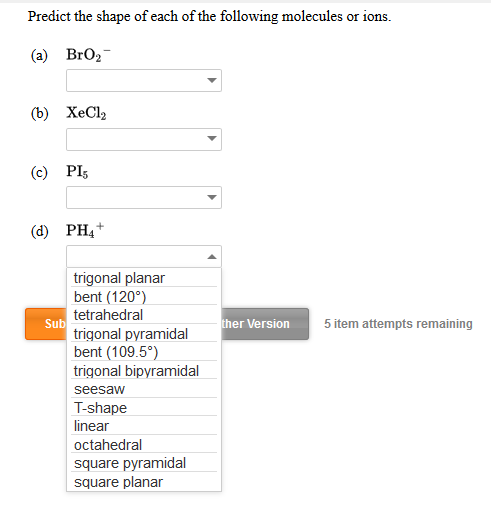Predict the shape of each of the following molecules or ions. (a) Br02 (b) XeCl2 (c) PIE (d) PH4+ Sub ther Version 5 item attempts remaining trigonal planar bent (120°) tetrahedral trigonal pyramidal bent (109.5°) trigonal bipyramidal seesaw T-shape linear octahedral square pyramidal square planar

Free Homework App